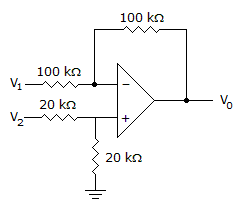# Electronic Devices - Op-Amp Applications - Discussion

Discussion Forum : Op-Amp Applications - General Questions (Q.No. 4)
4.
Determine the output voltage when V1 = –V2 = 1 V.0 V
–2 V
1 V
2 V
Explanation:
No answer description is available. Let's discuss.
Discussion:
28 comments Page 1 of 3.

Vaibhav said:   4 years ago
Simple and easy the circuit shown acts as a difference amplifier and output voltage is given by vo =( v2-v1)x(Rf/R1).
(4)

Praveen said:   4 years ago
Vo = (-RF/R1)V1 + (1+(RF/R1))V2'.
= -1 + (1+1)(20*V1/40),
= -2.
(3)

Shrestha said:   5 years ago
According to the differential amplifier fromula:

Vout = (R2/R1)*(V2-V1).
= (20/20)*(-1-1).
= 1*-2 = -2.
(2)

Avi said:   5 years ago
[R3/(R1+R3][(R2+R4)/R2][V1]-[V2 (R4/R2)] is the formula. So it is 0V.

David khasu said:   5 years ago
Here, we can use {Rf÷R1}*(v1-v2).

ALI said:   6 years ago
(20/20+20)(100+100/100)*V1-(100/100)V2 = V1-V2.

The resulting output voltage is seen to be the difference of the two input voltage.
(1)

Rakesh said:   6 years ago
Vo=(-100/100)V1+(20/(20+20))((100+100)/100)V2.
Vo=-V1+(1/2)(2)V2.
Vo=-V1+V2,
Vo=-1+(-1) because -V2=1 => V2=-1,
Vo=-2 Volts.

Sugin V said:   7 years ago
Here, V2/2 (1+Rf/Rs) + V1 (-Rf/Rs).

Surya said:   7 years ago
It is diff amplifier Vo = (v2-v1) = (-1-1) =-2.
(2)

Shawn said:   8 years ago
I think the answer should be 0 V.

Because the is not powered. So no amplification.